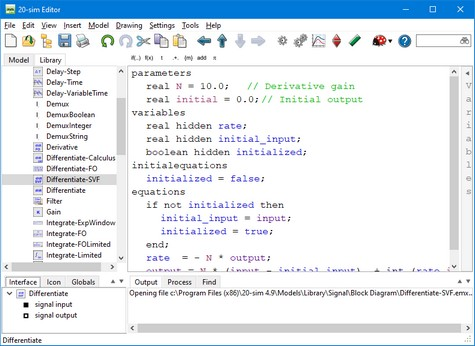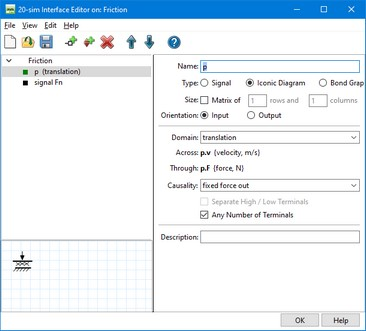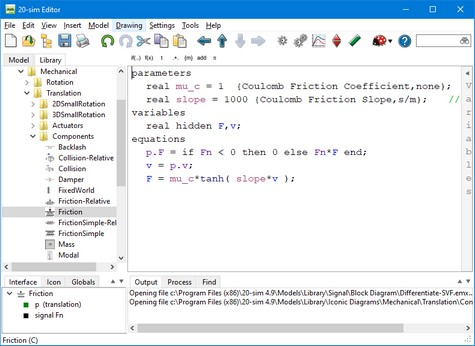﻿ 20-sim webhelp > Language Reference > Introduction > Using Port Names

# Using Port Names

To use port variables in equations, you must use the port names that are defined in the Interface Editor.

## Signal Ports

For signal ports, you can directly use the port names. The example below, shows the library model Differentiate-SVF.emx. In the Interface Editor, two ports are defined with the names input and output. In the equations these names are used.The Interface tab shows the contents of the Interface Editor.

## Power ports

Power ports describe the flow of power and are always characterized by two variables: the power-port variables. For bond graph ports the extensions .e and .f are used to indicate these variables. For iconic diagram ports many aliases are known, for example .u and .i for the electric domain. You can use these power-port variables in equations by typing the port name followed by a dot and the extension.

You can always find the correct extensions by opening the Interface Editor and selecting a port. The example below, shows the library model Friction.emx. In the Interface Editor, one power port is defined with the name p. As shown, the correct description of both variables is p.v and p.F.Double clicking in the Interface tab will open the Interface Editor.

The equation description of this model (see below) shows the use of the variables p.v and p.F.In the equations the port variables p.F and p.v are used.